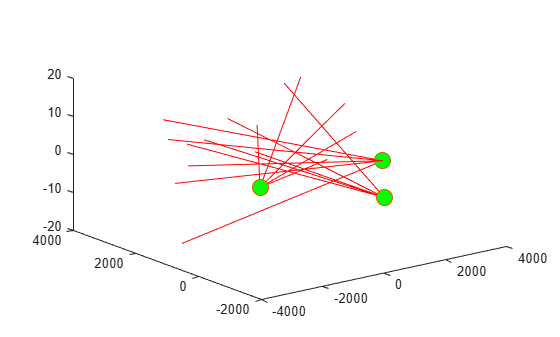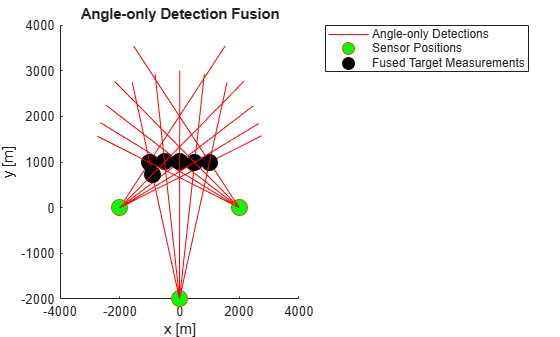# staticDetectionFuser

Static fusion of synchronous sensor detections

Since R2018b

## Description

`staticDetectionFuser` System object™ creates a static detection fuser object to fuse angle-only sensor detections.

To obtain the fuser:

1. Create the `staticDetectionFuser` object and set its properties.

2. Call the object with arguments, as if it were a function.

## Creation

### Syntax

``fuser = staticDetectionFuser()``
``fuser = staticDetectionFuser(Name,Value)``

### Description

````fuser = staticDetectionFuser()` creates a default three-sensor static detection fuser object to fuse angle-only sensor detections.```

example

````fuser = staticDetectionFuser(Name,Value)` sets properties using one or more name-value pairs. For example, ```fuser = staticDetectionFuser('FalseAlarmRate',1e-6,'MaxNumSensors',12)``` creates a fuser that has a maximum of 12 sensors and a false alarm rate of `1e-6`. Enclose each property name in quotes.```

## Properties

expand all

Unless otherwise indicated, properties are nontunable, which means you cannot change their values after calling the object. Objects lock when you call them, and the `release` function unlocks them.

If a property is tunable, you can change its value at any time.

Sensor index of the composite detections reported by the fuser, specified as a positive integer. This index becomes the `SensorIndex` of `objectDetection` objects returned by the fuser.

Example: `5`

Data Types: `double`

Function for fusing multiple sensor detections, specified as a character vector, string, or function handle. The function fuses multiple detections into one and returns the fused measurement and measurement noise. Any fusing function combines at most one detection from each sensor. The syntax of the measurement fuser function is:

`[fusedMeasurement,fusedMeasurementNoise] = MeasurementFusionFcn(detections)`
where the input and output functions arguments are

• `detections` – cell array of `objectDetection` measurements.

• `fusedMeasurement` – an N-by-1 vector of fused measurements.

• `fusedMeasurementNoise` – an N-by-N matrix of fused measurements noise.

The value of N depends on the `MeasurementFormat` property.

 MeasurementFormat Property N `'Position'` 1, 2, and 3 `'Velocity` 1, 2, and 3 `'PositionAndVelocity` 2, 4, and 6 `'Custom'` Any

Data Types: `char` | `string` | `function_handle`

Format of the fused measurement, specified as `'Position'`, `'Velocity'`, `'PositionAndVelocity'`, or `'Custom'`. The formats are

• `'Position'` – the fused measurement is the position of the target in the global coordinate frame.

• `'Velocity'` – the fused measurement is the velocity of the target in the global coordinate frame.

• `'PositionAndVelocity'` – the fused measurement is the position and velocity of the target in the global coordinate frame defined according to the format `[x;vx;y;vy;z;vz]`.

• `'Custom'` – custom fused measurement. To enable this format, specify a function using the `MeasurementFcn`.

Example: `'PositionAndVelocity'`

Custom measurement function, specified as a character vector, string, or function handle. Specify the function that transforms fused measurements into sensor measurements. The function must have the following signature:

`sensorMeas = MeasurementFcn(fusedMeas,measParameters)`

#### Dependencies

To enable this property, set the `MeasurementFormat` property to `'Custom'`.

Data Types: `char` | `string` | `function_handle`

Maximum number of sensors in surveillance region, specified as a positive integer greater than one.

Data Types: `double`

Volume of sensors detection bins, specified as a positive scalar or N-length vector of positive scalars. N is the number of sensors. If specified as a scalar, each sensor is assigned the same volume. If a sensor produces an angle-only measurement, for example, azimuth and elevation, the volume is defined as the solid angle subtended by one bin.

Data Types: `double`

Probability of detection of a target by each sensor, specified as a scalar or N-length vector of positive scalars in the range (0,1). N is the number of sensors. If specified as a scalar, each sensor is assigned the same detection probability. The probability of detection is used in calculating the cost of fusing a "one" (target was detected) or "zero" (target was not detected) detections from each sensor.

Example: `0.99`

Data Types: `double`

Rate at which false positives are reported by sensor in each bin, specified as a scalar or N-length vector of positive scalars. N is the number of sensors. If specified as a scalar, each sensor is assigned the same false alarm rate. The false alarm rate is used to calculate the likelihood of clutter in the detections reported by each sensor.

Example: `1e-5`

Data Types: `double`

Option to use parallel computing resources, specified as `false` or `true`. The `staticDetectionFuser` calculates the cost of fusing detections from each sensor as an n-D assignment problem. The fuser spends most of the time in computing the cost matrix for the assignment problem. If Parallel Computing Toolbox™ is installed, this option lets the fuser use the parallel pool of workers to compute the cost matrix.

Data Types: `logical`

Absolute tolerance between timestamps of detections, specified as a nonnegative scalar. The `staticDetectionFuser` assumes that sensors are synchronous. This property defines the allowed tolerance value between detection time-stamps to still be considered synchronous.

Example: `1e-3`

Data Types: `double`

## Usage

### Syntax

``compositeDets = fuser(dets)``
``[compositeDets,analysisInfo] = fuser(dets)``

### Description

````compositeDets = fuser(dets)` returns the fused detections, `compositeDets`, of input detections, `dets`.```
````[compositeDets,analysisInfo] = fuser(dets)` also returns analysis information, `analysisInfo`.```

### Input Arguments

expand all

Pre-fused detections, specified as a cell array of `objectDetection` objects.

### Output Arguments

expand all

Pre-fused detections, returned as a cell array of `objectDetection` objects.

Analysis information, returned as a structure. The fields of the structure are:

• `CostMatrix`N-dimensional cost matrix providing the cost of association of detections, where N is the number of sensors. The cost is the negative log-likelihood of the association and can be interpreted as the negative score of the track that will be generated by the fused measurement.

• `Assignments` – A P-by-N list of assignments, where P is the number of composite detections.

• `FalseAlarms` – A Q-by-1 list of indices of detections declared as false alarms by association.

Data Types: `struct`

## Object Functions

To use an object function, specify the System object as the first input argument. For example, to release system resources of a System object named `obj`, use this syntax:

`release(obj)`

expand all

 `release` Release resources and allow changes to System object property values and input characteristics `reset` Reset internal states of System object `isLocked` Determine if System object is in use `clone` Create duplicate System object

## Examples

collapse all

Fuse angle-only detections from three ESM sensors.

Load stored detections from the sensors.

`load('angleOnlyDetectionFusion.mat','detections');`

Visualize angle-only detections for plotting the direction vector.

```rPlot = 5000; plotData = zeros(3,numel(detections)*3); for i = 1:numel(detections) az = detections{i}.Measurement(1); el = detections{i}.Measurement(2); [xt,yt,zt] = sph2cart(deg2rad(az),deg2rad(el),rPlot); % The sensor is co-located at platform center, therefore use % the position from the second measurement parameter originPos = detections{i}.MeasurementParameters(2).OriginPosition; positionData(:,i) = originPos(:); plotData(:,3*i-2) = [xt;yt;zt] + originPos(:); plotData(:,3*i-1) = originPos(:); plotData(:,3*i) = [NaN;NaN;NaN]; end plot3(plotData(1,:),plotData(2,:),plotData(3,:),'r-') hold on plot3(positionData(1,:),positionData(2,:),positionData(3,:),'o','MarkerSize',12,'MarkerFaceColor','g')```Create a `staticDetectionFuser` to fuse angle-only detections using the measurement fusion function `triangulateLOS`.

`fuser = staticDetectionFuser('MeasurementFusionFcn','triangulateLOS','MaxNumSensors',3)`
```fuser = staticDetectionFuser with properties: FusedSensorIndex: 1 MeasurementFusionFcn: 'triangulateLOS' MeasurementFormat: 'Position' MaxNumSensors: 3 Volume: [3x1 double] DetectionProbability: [3x1 double] FalseAlarmRate: [3x1 double] TimeTolerance: 1.0000e-06 UseParallel: false ```

Create the fused detections and obtain the analysis information.

```[fusedDetections, analysisInfo] = fuser(detections); fusedPositions = zeros(3,numel(fusedDetections)); for i = 1:numel(fusedDetections) fusedPositions(:,i) = fusedDetections{i}.Measurement; end plot3(fusedPositions(1,:),fusedPositions(2,:),fusedPositions(3,:),'ko', ... 'MarkerSize',12, 'MarkerFaceColor','k') legend('Angle-only Detections','Sensor Positions','Fused Target Measurements') title('Angle-only Detection Fusion') xlabel('x [m]') ylabel('y [m]') view(2)```Use the `analysisInfo` output to check the assignments.

`analysisInfo.Assignments`
```ans = 6x3 uint32 matrix 0 10 14 1 6 11 2 7 12 3 8 13 4 9 0 5 0 15 ```

## Algorithms

expand all

 Bar-Shalom, Yaakov, Peter K. Willett, and Xin Tian. Tracking and data fusion. Storrs, CT, USA:: YBS publishing, 2011.